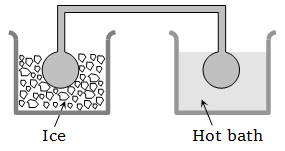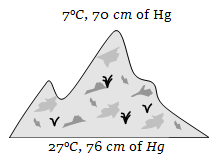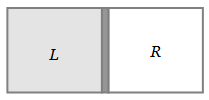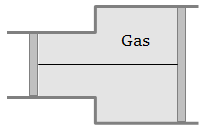Two identical glass bulbs are interconnected by a thin glass tube. A gas is filled in these bulbs at N.T.P. If one bulb is placed in ice and another bulb is placed in hot bath, then the pressure of the gas becomes 1.5 times. The temperature of hot bath will be1.  100°C

2.  182°C

3.  256°C

4.  546°C

High Yielding Test Series + Question Bank - NEET 2020

Difficulty Level:

Two containers of equal volume contain the same gas at pressures ${\mathrm{P}}_{1}$ and ${\mathrm{P}}_{2}$ and absolute temperatures ${\mathrm{T}}_{1}$ and ${\mathrm{T}}_{2}$ respectively. On joining the vessels, the gas reaches a common pressure P and common temperature T. The ratio P/T is equal to

1. $\frac{{\mathrm{P}}_{1}}{{\mathrm{T}}_{1}}+\frac{{\mathrm{P}}_{2}}{{\mathrm{T}}_{2}}$

2. $\frac{{\mathrm{P}}_{1}{\mathrm{T}}_{1}+{\mathrm{P}}_{2}{\mathrm{T}}_{2}}{{\left({\mathrm{T}}_{1}+{\mathrm{T}}_{2}\right)}^{2}}$

3. $\frac{{\mathrm{P}}_{1}{\mathrm{T}}_{2}+{\mathrm{P}}_{2}{\mathrm{T}}_{1}}{{\left({\mathrm{T}}_{1}+{\mathrm{T}}_{2}\right)}^{2}}$

4. $\frac{{\mathrm{P}}_{1}}{2{\mathrm{T}}_{1}}+\frac{{\mathrm{P}}_{2}}{2{\mathrm{T}}_{2}}$

High Yielding Test Series + Question Bank - NEET 2020

Difficulty Level:

At the top of a mountain a thermometer reads 7°C and a barometer reads 70 cm of Hg. At the bottom of the mountain these read 27°C and 76 cm of Hg respectively. Comparison of density of air at the top with that of bottom is1.  75/76

2.  70/76

3.  76/75

4.  76/70

High Yielding Test Series + Question Bank - NEET 2020

Difficulty Level:

The root mean square speed of the molecules of a diatomic gas is v. When the temperature is doubled, the molecules dissociate into two atoms. The new root mean square speed of the atom is

1.  $\sqrt{2}\mathrm{v}$

2.  $\mathrm{v}$

3.  $2\mathrm{v}$

4.  $4\mathrm{v}$

High Yielding Test Series + Question Bank - NEET 2020

Difficulty Level:

A vessel is partitioned in two equal halves by a fixed diathermic separator. Two different ideal gases are filled in left (L) and right (R) halves. The rms speed of the molecules in L part is equal to the mean speed of molecules in the R part. Then the ratio of the mass of a molecule in L part to that of a molecule in R part is1. $\sqrt{\frac{3}{2}}$

2. $\sqrt{\frac{\mathrm{\pi }}{4}}$

3. $\sqrt{\frac{2}{3}}$

4. $\frac{3\mathrm{\pi }}{8}$

High Yielding Test Series + Question Bank - NEET 2020

Difficulty Level:

A gas is filled in the cylinder shown in the figure. The two pistons are joined by a string. If the gas is heated, the pistons will1.Move towards left

2. Move towards right

3. Remain stationary

4. None of these

High Yielding Test Series + Question Bank - NEET 2020

Difficulty Level:

A closed vessel contains 8gm of oxygen and 7gm of nitrogen. The total pressure is 10 atm at a given temperature. If now oxygen is absorbed by introducing a suitable absorbent the pressure of the remaining gas in atm will be

1.  2

2.  10

3.  4

4.  5

High Yielding Test Series + Question Bank - NEET 2020

Difficulty Level:

${\mathrm{CO}}_{2}\left(\mathrm{O}=\mathrm{C}=\mathrm{O}\right)$ is a triatomic gas. Mean kinetic energy of one gram gas will be (If N-Avogadro's number, k-Boltzmann's constant and molecular weight of ${\mathrm{CO}}_{2}=44$ , Degree of freedom f = 7)

1.

2.

3.

4.

High Yielding Test Series + Question Bank - NEET 2020

Difficulty Level:

40 calories of heat is needed to raise the temperature of 1 mole of an ideal monoatomic gas from 20°C to 30°C at a constant pressure. The amount of heat required to raise its temperature over the same interval at a constant volume  is

1.  20 calorie

2.  40 calorie

3.  60 calorie

4.  80 calorie

High Yielding Test Series + Question Bank - NEET 2020

Difficulty Level:

The pressure and volume of saturated water vapour are P and V respectively. It is compressed isothermally thereby volume becomes V/2, the final pressure will be

1.  More than 2P

2.  P

3.  2P

4.  4P